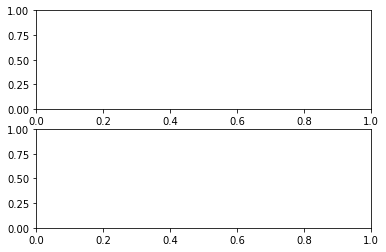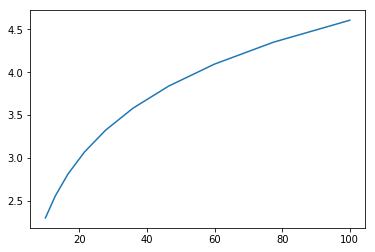We are working to support a site-wide PDF but it is not yet available. You can download PDFs for individual lectures through the download badge on each lecture page.

Code should execute sequentially if run in a Jupyter notebook

Debugging¶

Contents¶

“Debugging is twice as hard as writing the code in the first place. Therefore, if you write the code as cleverly as possible, you are, by definition, not smart enough to debug it.” – Brian Kernighan

Overview¶

Are you one of those programmers who fills their code with print statements when trying to debug their programs?

Hey, we all used to do that

(OK, sometimes we still do that…)

But once you start writing larger programs you’ll need a better system

Debugging tools for Python vary across platforms, IDEs and editors

Here we’ll focus on Jupyter and leave you to explore other settings

We’ll need the following imports

In :
import numpy as np
import matplotlib.pyplot as plt
%matplotlib inline


Debugging¶

The debug Magic¶

Let’s consider a simple (and rather contrived) example

In :
def plot_log():
fig, ax = plt.subplots(2, 1)
x = np.linspace(1, 2, 10)
ax.plot(x, np.log(x))
plt.show()

plot_log()  # Call the function, generate plot

---------------------------------------------------------------------------
AttributeError                            Traceback (most recent call last)
<ipython-input-2-c32a2280f47b> in <module>()
5     plt.show()
6
----> 7 plot_log()  # Call the function, generate plot

<ipython-input-2-c32a2280f47b> in plot_log()
2     fig, ax = plt.subplots(2, 1)
3     x = np.linspace(1, 2, 10)
----> 4     ax.plot(x, np.log(x))
5     plt.show()
6

AttributeError: 'numpy.ndarray' object has no attribute 'plot'This code is intended to plot the log function over the interval $[1, 2]$

But there’s an error here: plt.subplots(2, 1) should be just plt.subplots()

(The call plt.subplots(2, 1) returns a NumPy array containing two axes objects, suitable for having two subplots on the same figure)

The traceback shows that the error occurs at the method call ax.plot(x, np.log(x))

The error occurs because we have mistakenly made ax a NumPy array, and a NumPy array has no plot method

But let’s pretend that we don’t understand this for the moment

We might suspect there’s something wrong with ax but when we try to investigate this object, we get the following exception:

In :
ax

---------------------------------------------------------------------------
NameError                                 Traceback (most recent call last)
<ipython-input-3-b00e77935981> in <module>()
----> 1 ax

NameError: name 'ax' is not defined

The problem is that ax was defined inside plot_log(), and the name is lost once that function terminates

Let’s try doing it a different way

We run the first cell block again, generating the same error

In :
def plot_log():
fig, ax = plt.subplots(2, 1)
x = np.linspace(1, 2, 10)
ax.plot(x, np.log(x))
plt.show()

plot_log()  # Call the function, generate plot

---------------------------------------------------------------------------
AttributeError                            Traceback (most recent call last)
<ipython-input-4-c32a2280f47b> in <module>()
5     plt.show()
6
----> 7 plot_log()  # Call the function, generate plot

<ipython-input-4-c32a2280f47b> in plot_log()
2     fig, ax = plt.subplots(2, 1)
3     x = np.linspace(1, 2, 10)
----> 4     ax.plot(x, np.log(x))
5     plt.show()
6

AttributeError: 'numpy.ndarray' object has no attribute 'plot'But this time we type in the following cell block

%debug


You should be dropped into a new prompt that looks something like this

ipdb>


Now we can investigate the value of our variables at this point in the program, step forward through the code, etc.

For example, here we simply type the name ax to see what’s happening with this object:

ipdb> ax
array([<matplotlib.axes.AxesSubplot object at 0x290f5d0>,
<matplotlib.axes.AxesSubplot object at 0x2930810>], dtype=object)


It’s now very clear that ax is an array, which clarifies the source of the problem

To find out what else you can do from inside ipdb (or pdb), use the online help

ipdb> h

Documented commands (type help <topic>):
========================================
EOF    bt         cont      enable  jump  pdef   r        tbreak   w
a      c          continue  exit    l     pdoc   restart  u        whatis
alias  cl         d         h       list  pinfo  return   unalias  where
args   clear      debug     help    n     pp     run      unt
b      commands   disable   ignore  next  q      s        until
break  condition  down      j       p     quit   step     up

Miscellaneous help topics:
==========================
exec  pdb

Undocumented commands:
======================
retval  rv

ipdb> h c
c(ont(inue))
Continue execution, only stop when a breakpoint is encountered.


Setting a Break Point¶

The preceding approach is handy but sometimes insufficient

Consider the following modified version of our function above

In :
def plot_log():
fig, ax = plt.subplots()
x = np.logspace(1, 2, 10)
ax.plot(x, np.log(x))
plt.show()

plot_log()Here the original problem is fixed, but we’ve accidentally written np.logspace(1, 2, 10) instead of np.linspace(1, 2, 10)

Now there won’t be any exception, but the plot won’t look right

To investigate, it would be helpful if we could inspect variables like x during execution of the function

To this end, we add a “break point” by inserting breakpoint() inside the function code block

def plot_log():
breakpoint()
fig, ax = plt.subplots()
x = np.logspace(1, 2, 10)
ax.plot(x, np.log(x))
plt.show()

plot_log()


Now let’s run the script, and investigate via the debugger

> <ipython-input-6-a188074383b7>(6)plot_log()
-> fig, ax = plt.subplots()
(Pdb) n
> <ipython-input-6-a188074383b7>(7)plot_log()
-> x = np.logspace(1, 2, 10)
(Pdb) n
> <ipython-input-6-a188074383b7>(8)plot_log()
-> ax.plot(x, np.log(x))
(Pdb) x
array([ 10.        ,  12.91549665,  16.68100537,  21.5443469 ,
27.82559402,  35.93813664,  46.41588834,  59.94842503,
77.42636827, 100.        ])


We used n twice to step forward through the code (one line at a time)

Then we printed the value of x to see what was happening with that variable

To exit from the debugger, use q

Other Useful Magics¶

In this lecture we used the %debug IPython magic

There are many other useful magics:

• %precision 4 sets printed precision for floats to 4 decimal places
• %whos gives a list of variables and their values
• %quickref gives a list of magics

The full list of magics is here

• Share page